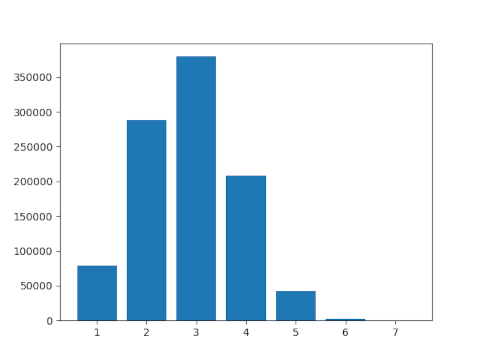# Numbers don’t typically have many prime factors

Suppose you select a 100-digit number at random. How many distinct prime factors do you think it would have?

The answer is smaller than you might think: most likely between 5 and 6.

The function ω(n) returns the number of distinct prime factors of n . A theorem of Hardy and Ramanujan says that as N goes to infinity, the average value of ω(n) for positive integers up to N is log log N.

Since log log 10100 = 5.43…, we’d expect 100-digit numbers to have between 5 and 6 distinct factors.

The above calculation gives the average number of distinct prime factors for all numbers with up to 100 digits. But if we redid the calculation looking at numbers between 1099 and 10100 it would only make a difference in the third decimal place.

Let’s look at a much smaller example where we can tally the values of ω(n), numbers from 2 to 1,000,000.Most numbers with up to six digits have two or three distinct prime factors, which is consistent with log log 106 ≈ 2.6.

There are 2,285 six-digit numbers that have six distinct prime factors. Because this is out of a million numbers, it corresponds to a bar in the graph above that is barely visible.

There are 8 six-digit number with 7 distinct prime factors and none with more factors.

Hardy’s theorem with Ramanujan established the mean of ω(n) for large numbers. The Erdős–Kac theorem goes further and says roughly that ω(n) is normally distributed with mean and variance log log n. More on this here.

So returning to our example of 100-digit numbers, the Hardy-Ramanujan theorem implies these numbers would have between 5 and 6 prime factors on average, and the Erdős–Kac theorem implies that about 95% of such numbers have 10 or fewer distinct prime factors. The maximum number of distinct prime factors is 54.

## Related posts

 SymPy has a function `primeomega`, but it does not compute ω(n). Instead, it computes Ω(n), the number of prime factors of n counted with multiplicity. So, for example, ω(12) = 2 but Ω(12) = 3. The SymPy function to compute ω(n) is called `primenu`.

## One thought on “Numbers don’t typically have many prime factors”

1. Jonathan

It’s not an exact correspondence, but it feels like this result shares some of the spirit of the observation that “99% of groups of order less than 2000 are of order 1024”, which generalizes to “almost all groups are of order \$2^n\$”